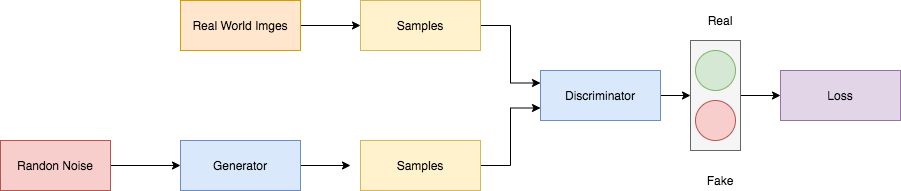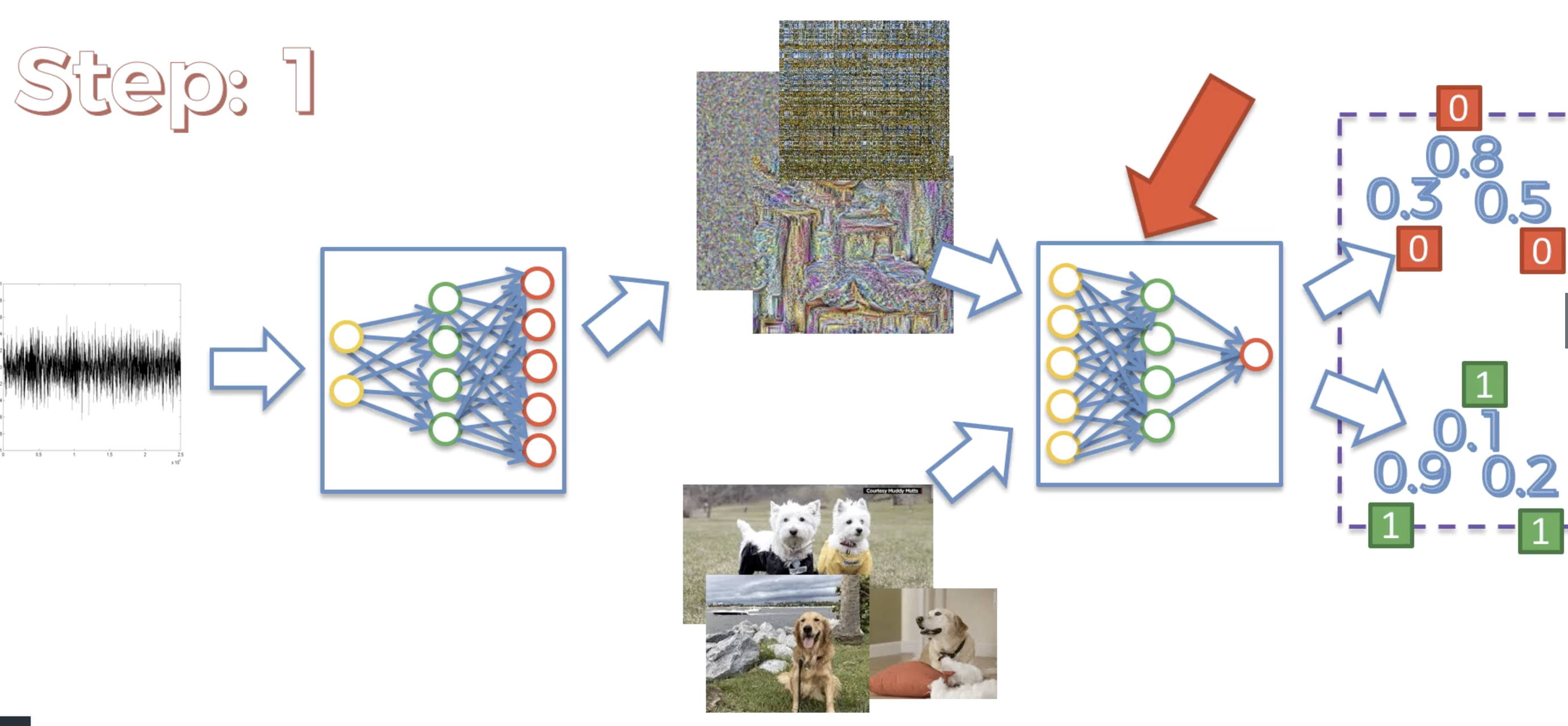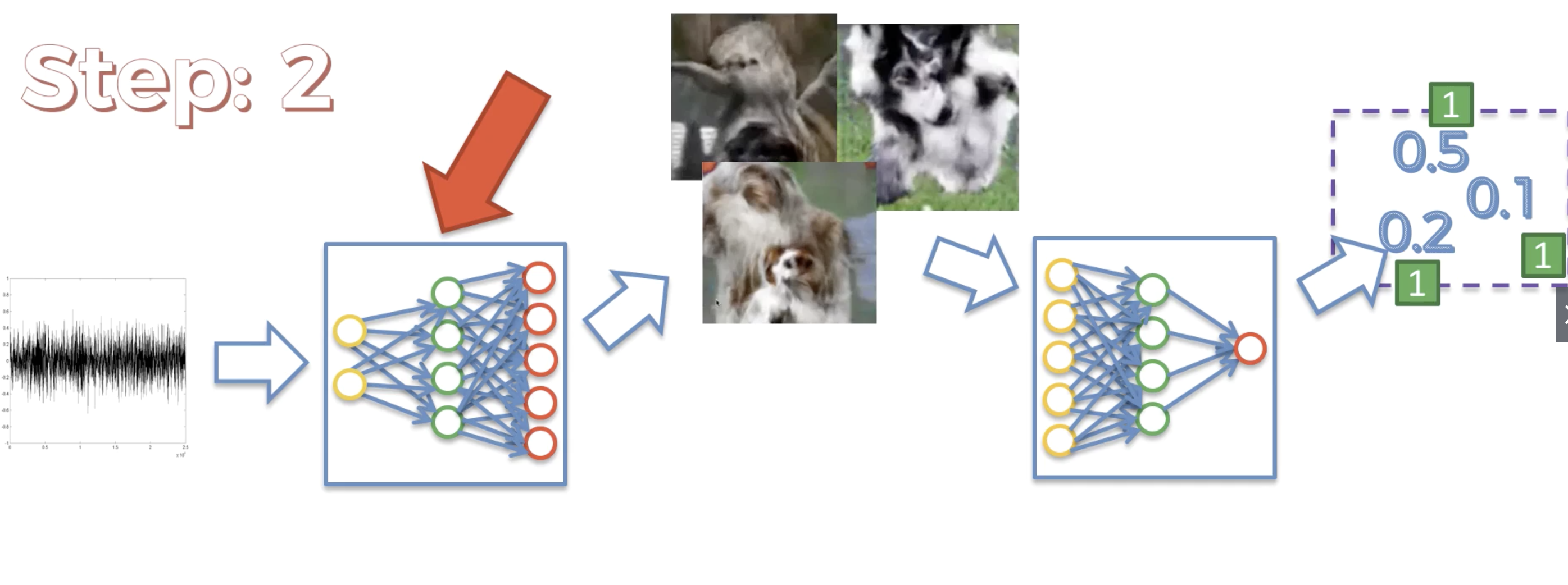Related Articles
Basics of Generative Adversarial Networks (GANs)
• Last Updated : 18 Jul, 2020

GANs is an approach for generative modeling using deep learning methods such as CNN (Convolutional Neural Network). Generative modeling is an unsupervised learning approach that involves automatically discovering and learning patterns in input data such that the model can be used to generate new examples from the original dataset.

GANs is a way of training a generative model by framing problem as a supervised learning problem with two sub-models. There are two components of GANs:

1. Generator: It is trained to generate new dataset, for example in computer vision it generates new images from existing real world images.
2. Discriminator: It compares those images with some real world examples and classify real and fake images.

Example:
The Generator generates some random images (eg. tables) and then the discriminator compares those images with some real world table images and sends the feed back to itself and Generator. Look at GAN structure in fig. 1.Fig. 1: GAN structure

How does GAN works? Let’s take an example of generating images of Dogs.

Step 1- Training of Discriminator

1. Firstly some random noise signal is sent to a generator which creates some useless images containing noise
2. (See fig. 2)

.

3. Two inputs are given to Discriminator. First is the sample output images generated from Generator and second being the real world dog image samples.
4. There after, The Discriminator populates some values (probability) after comparing both the images which can be seen in fig. 2. It calculates 0.8, 0.3 and 0.5 for generator output images and 0.1, 0.9 and 0.2 for the real world images.
5. Now, an error is calculated by comparing probabilities of generated images with 0 (Zero) and comparing probabilities of real-word images with 1 (One). (Ex. 0-0.8, 0-0.3, 0-0.5 and 1-0.1, 1-0.9, 1-0.2).
6. After calculating individual errors, it will calculate cumulative error(loss) which is backpropagated and the weights of the Discriminator are adjusted. This is how a Discriminator is trained.Fig. 2: GAN Step 1

Step 2 – Training of Generator:
1. As previously in step 1, the loss is back propagated to discriminator to adjust its weights. Now we also need to back propagate an error to the Generator so that it can adjust its weights as well and train itself to generate improved images.
2. Now, the images generated from the Generator are used as input to the generator itself instead of random noise.
3. The newly generated images from the generator will now be an input to the Discriminator which again calculates probabilities like 0.5, 0.1 and 0.2. (See fig. 2)
4. Now, an error will be calculated by comparing probabilities of generated images with 1(One).
5. After calculating individual errors, it will calculate cumulative error(loss) which is back propagated and the weights of Generator are adjusted. This is how Generator is trained.Fig. 2: GAN Step 2

After a few iterations, you will see that the Generator starts generating images close to real-world images.

Applications of GAN:

1. Generating Images
2. Super Resolution
3. Image Modification
4. Photo realistic images
5. Face AgeingMy Personal Notes arrow_drop_up